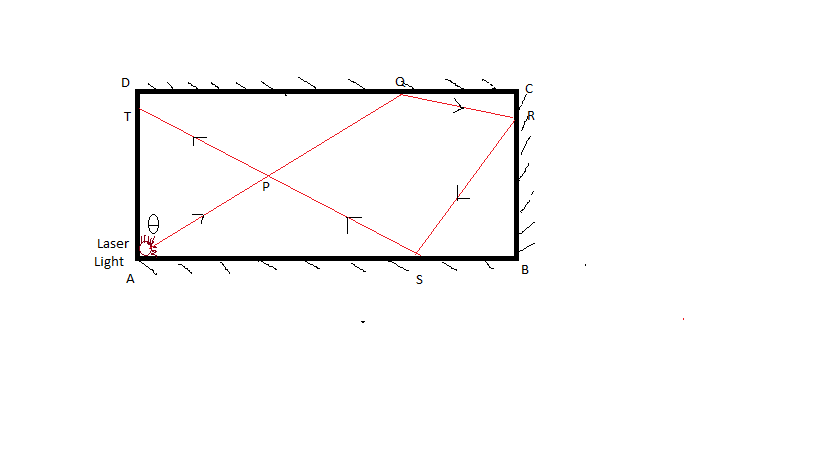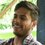# Optical Geometry : "Fun with Laser Light"Consider an rectangular glass board $ABCD$ , with surfaces $AB$, $BC$, $CD$ are perfectly silvered. An small laser light is set in the corner $A$ (negligible dimensions) . Now just for fun , this laser light is set in such a way so that light ray first travel from A making an angle $\theta$ with side $AD$ and then totally reflected by surfaces $DC$ , then $CB$ , then $BA$ and finally refracted out from surface $AD$. If during this event , an quadrilateral and an triangle are traced out as shown & their point of intersection is $P$, Such that Area of triangle( $APT$ ) is equal to that of quadrilateral( $PQRS$ ).

Given that :

$AB=CD=2(BC)=2(AD)$

$\text{Laws of reflection holds perfectly}$.

Then find that particular angle $\theta$ in degrees or just simply prove that $\tan { \theta } =\cfrac { a-\sqrt { b } }{ c }$ and find $a+b+c$ , for which such event is possible.Note by Deepanshu Gupta
5 years, 10 months ago

This discussion board is a place to discuss our Daily Challenges and the math and science related to those challenges. Explanations are more than just a solution — they should explain the steps and thinking strategies that you used to obtain the solution. Comments should further the discussion of math and science.

When posting on Brilliant:

• Use the emojis to react to an explanation, whether you're congratulating a job well done , or just really confused .
• Ask specific questions about the challenge or the steps in somebody's explanation. Well-posed questions can add a lot to the discussion, but posting "I don't understand!" doesn't help anyone.
• Try to contribute something new to the discussion, whether it is an extension, generalization or other idea related to the challenge.
• Stay on topic — we're all here to learn more about math and science, not to hear about your favorite get-rich-quick scheme or current world events.

MarkdownAppears as
*italics* or _italics_ italics
**bold** or __bold__ bold
- bulleted- list
• bulleted
• list
1. numbered2. list
1. numbered
2. list
Note: you must add a full line of space before and after lists for them to show up correctly
paragraph 1paragraph 2

paragraph 1

paragraph 2

[example link](https://brilliant.org)example link
> This is a quote
This is a quote
    # I indented these lines
# 4 spaces, and now they show
# up as a code block.

print "hello world"
# I indented these lines
# 4 spaces, and now they show
# up as a code block.

print "hello world"
MathAppears as
Remember to wrap math in $$ ... $$ or $ ... $ to ensure proper formatting.
2 \times 3 $2 \times 3$
2^{34} $2^{34}$
a_{i-1} $a_{i-1}$
\frac{2}{3} $\frac{2}{3}$
\sqrt{2} $\sqrt{2}$
\sum_{i=1}^3 $\sum_{i=1}^3$
\sin \theta $\sin \theta$
\boxed{123} $\boxed{123}$

## Comments

Sort by:

Top Newest

I get Tan(theta)= 4-√8 Can someone confirm

- 2 years, 9 months ago

Log in to reply

×

Problem Loading...

Note Loading...

Set Loading...